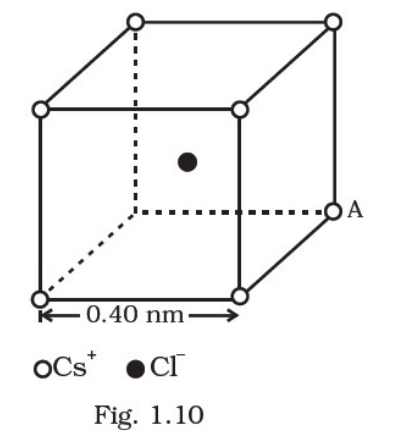Deepak Scored 45->99%ile with Bounce Back Crack Course. You can do it too!

# represents a crystal unit of cesium chloride,

Question:

represents a crystal unit of cesium chloride, CsCl. The cesium atoms, represented by open circles are situated at the corners of a cube of side 0.40 nm, whereas a Cl atom is situated at the centre of the cube. The Cs atoms are deficient in one electron while the Catom carries an excess electron.(i) What is the net electric field on the Cl atom due to eight Cs atoms?

(ii) Suppose that the Cs atom at the corner A is missing. What is the net force now on the Catom due to seven remaining Cs atoms?

Solution:

(i) From the given figure, we can observe that Cl atom is at the centre whereas Cs+ atoms are at eight corners at equal distance. So, by symmetry net force on Cl atom due to other Cs+ atoms is zero. Due to which, net electric field due to other Cs+ atoms is also zero (as, |E| = F/q)

(ii) In the given question, removing Cs atom at the corner A is equivalent to adding a singly charged negative Cs ion at point A.

So,

Net force = q2/(4πεor2)

Here, q = charge on electron, r = distance between Cl and Cs atoms

Applying Pythagoras theorem, we have

$r=\sqrt{(0.02)^{2}+(0.20)^{2}+(0.20)^{2}} \times 10^{-9} \mathrm{~m}=0.346 \times 10^{-9} \mathrm{~m}$

Now,

Net force $=\frac{q^{2}}{4 \pi \varepsilon_{0} r^{2}}=\frac{9 \times 10^{9}\left(1.6 \times 10^{-19}\right)^{2}}{\left(0.346 \times 10^{-9}\right)^{2}}=1.92 \times 10^{-9} \mathrm{~N}$

The direction of force is from A to Cl